4132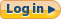| Help# Lesson 2 - Profit and Loss Graph

by Uncle Bob Williams

The Profit / Loss Graph is the key tool used to evaluate Options Trades, and it's easy to understand. It is important to take a minute to examine the graph, so we can easily understand what the potential Profit and Loss is of our various Options positions.

(1) The Left side of the graph (The Y or Vertical Axis) shows us the AMOUNT OF PROFIT and LOSS: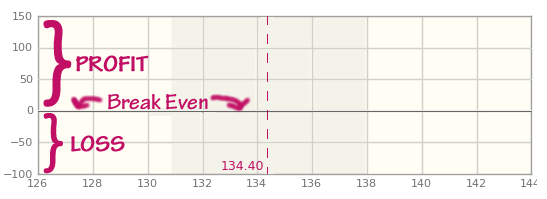The \$0 or Break Even line is in the middle. Anything above the Break Even line is where we make a Profit, anything below the Break Even line is where we have a loss.

(2) The Bottom side of the graph (The X or Horizontal Axis) shows us the PRICE OF THE STOCK / INDEX: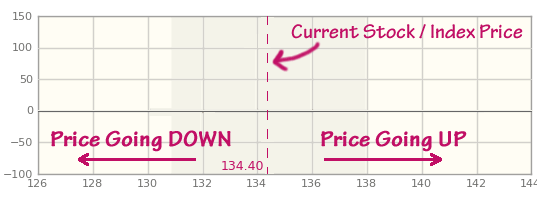The Current price is near the middle.

(3) Here is an example if we bought a SHARE OF STOCK at the Current price.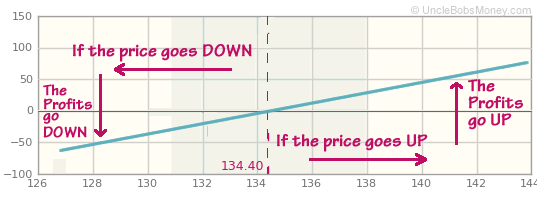The Blue line shows the Profit / Loss of our Stock as the price of the Stock goes up and down.

If the Price of our Stock goes UP, our Profit goes UP.

If the Price of our Stock goes DOWN, our Profit goes DOWN. (We have incurred a loss.)

(4) Now that we understand how the Profit / Loss Graph works, let's show an example using an Option:

=> We buy a CALL Option at the Strike of the Current Market Price. CALL Options make money when the Price goes UP.

=> If the price of the Stock goes DOWN, we only lose what we paid for this Option.

=> If the price of the Stock goes UP, we start making profits, and we start making profits like a rocket heading for space.

=> Note the Break Even Point, that is where the value of the Option equals what we paid for it. If the price of the Stock keeps going up, we will start making significant profits fast.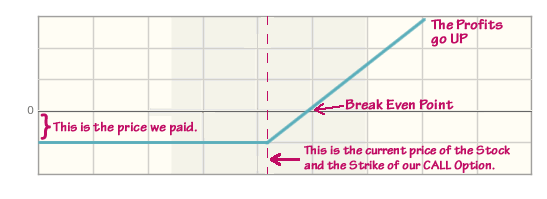Have a Question? Comment? Suggestion? Ask us.